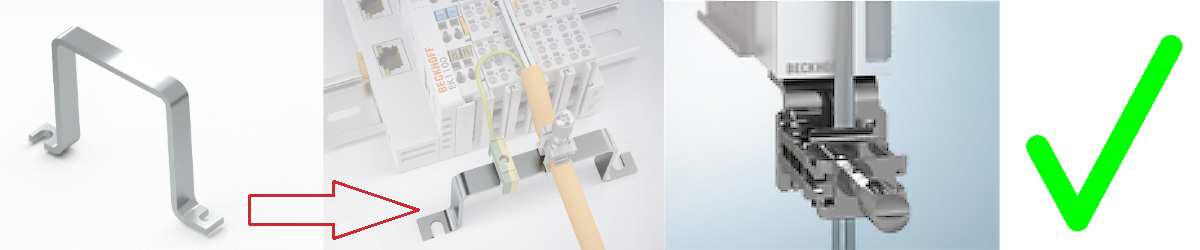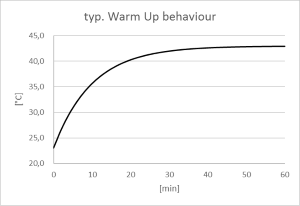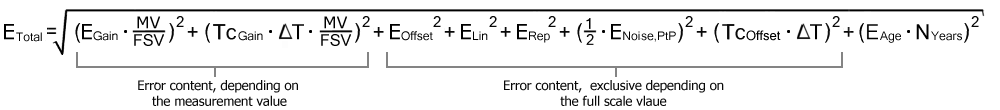# General information on measuring accuracy/measurement uncertainty

For basic information regarding the explanatory notes below, please refer to chapter "Notes on analog measured values", particularly full scale value.

This guidance could be worth to read for saving effort, time and presumably money, too.

Accurate knowledge of these instructions can give you the ease of mastering the technique in all applications and thus facilitate your work.

## Basic information on measurement technology:

Using measuring devices an attempt is made with a greater or lesser degree of expenditure to determine the "true value" of a measured variable, for example the ambient temperature. For various practical reasons this is not conclusively possible. Depending on the expenditure, the measurement/measured value is subject to a random measuring error that cannot be eliminated. With its practically determined specification data, Beckhoff provides an approach with which the residual measurement uncertainty can theoretically be calculated in the individual case. The following paragraphs serve this purpose.

## General notes

No special maintenance required, although an annual inspection is recommended for the box.

If a factory calibration certificate is available for the device, a recalibration interval recommendation of 1 year applies, unless otherwise specified.

## Notes regarding the specification data:

• Measurement specifications are usually specified as "% of nominal full scale value" = “% full scale value (%FSV)”, unless otherwise specified.
• In conjunction with each individual value "typical" means that on average this parameter has the specified value. For individual box modules the parameter may deviate from the typical value. Once example is the current consumption.
• In the context of a limit (parameter is typically max./min. X) or with two limits (parameter is typically between X and Y) "typical" means that this parameter tends to be between the limits for individual box modules. Deviations are possible, however; see confidence level. Once example is noise. Usually no measurements are made, in order to be able to make statements about standard deviations or result frequencies. A typical value is usually indicated as such after the unit.
• The confidence level is 95%, unless otherwise specified.
• When operating in EMC-disturbed environments, twisted and shielded signal cables, grounded at least at one end, must be used in order to comply with the specification. The use of Beckhoff shielding accessories ZB8511 or ZS9100-0002 is recommended:The ZB8520 DIN rail fastening is not recommended with regard to the analog protective effect:• If not other specified, measurement errors etc. will be stated in electrical DC operation (no use of AC values). Measurement of an AC value influences the frequency slope of the analog input and therefore the measurement itself.

## Note on the temperature

The temperature within/outside the device affects the measurement through the electronics. A measuring setup is generally characterized by a temperature dependence, which is specified in the form of a temperature drift, for example. The specifications are based on a constant ambient temperature. Variable conditions (e.g. heating of the control cabinet, sudden temperature drop due to opening of the control cabinet in cold weather) resulting in a temperature change may alter the measured values through dynamic and heterogeneous temperature distribution. To rectify such effects, the temperature inside the device can be read online from the CoE and used for compensation. Some devices indicate electrically that they have thermally stabilized as well; see diagnosis features.

## The specification data apply:

• after a warm-up time under operating voltage and in fieldbus mode of least 60 minutes at constant ambient temperature
• Practical note: after power-on the device already warms up exponentially such that the major part of the warming depending on the device within a short time of approximately 10 to 15 minutes is passing through and the measuring characteristics moving within the specification limits.
• For clarification: typical trend of an inner temperature (no significance for a particular device):• Some devices displays by the CoE object 0xF900:02 that they are thermally stabilized and ∆T within the device is very small. This can be evaluated by application,
• in horizontal installation position, taking account of the minimum distances,
• at free convection (no forced ventilation),
• provided the specification data are adhered to.

Under different conditions, a user-specific compensation is required.

## Notes on calculation with the specification data:

The independent specification data can be divided into two groups:

• the data on offset/gain deviation, non-linearity, repeatability whose effect on the measurement cannot be influenced by the user. These are summarized by Beckhoff according to the calculation below to the so-called "basic accuracy at 23°C".
• the specification data whose effect on the measurement can be influenced by the user, namely
• the noise: Effect influenced by sample rate, filtering and
• the temperature: Effect influenced by air conditioning, shielding, control cabinet cooling, ...

The independent individual accuracy data are to be added quadratically according to the formula below in order to determine a total measurement accuracy - if there are no special conditions that that contradict a uniform distribution and thus the quadratic approach (RSS - root of the sum of the squares).

For measurement ranges where the temperature coefficient is given as TcTerminal only:

 EOffset : Offset specification (at 23°C) EGain : Gain/scale specification (at 23°C) ENoise, PtP : Noise specification as a peak-to-peak value (applies to all temperatures) MV : Measured value FSV : Full scale value ELin : Non-linearity error over the entire measuring range (applies to all temperatures) ERep : Repeatability (applies to all temperatures) TcOffset : Temperature coefficient offset TcGain : Temperature coefficient gain TcTerminal : Temperature coefficient of the box ΔT : Difference between the ambient temperature and the specified basic temperature (23°C unless specified otherwise) EAge : Error coefficient of ageing NYears : Number of years ETotal : Theoretical calculated total error

Let's say, for example, we have the following values by a determined measurement value of 8.13 V and 10 V measurement mode (FSV = 10 V) and NYears = 0:

• Gain specification: EGain = 60 ppm
• Offset specification: EOffset = 70 ppmFSV
• Non-linearity: ELin = 25 ppmFSV
• Repeatability: ERep = 20 ppmFSV
• Noise (without filtering): ENoise, PtP = 100 ppmpeak-to-peak
• Temperature coefficients:
• TcGain = 8 ppm/K
• TcOffset = 5 ppmFSV/K

Then the theoretical possible total measurement accuracy at ΔT = 12K to the basic temperature can be calculated as follows:

or = ±0.0143.. %FSV

 Remarks: ppm ≙ 10-6 % ≙ 10-2

In general, you can calculate as follows:

• If only the application at 23°C is to be considered:
Total measurement accuracy = basic accuracy & noise according to above formula
• If the application at 23°C is to be considered with slow measurement (=average value formation/filtering):
Total measurement accuracy = basic accuracy
• If the general use is to be considered with known temperature range and incl. noise:
Total measurement accuracy = basic accuracy & noise & temperature values according to above formula

Beckhoff usually gives the specification data symmetrically in [±%], i.e. for example ±0.01% or ±100 ppm. Accordingly, therefore, the unsigned total window would be double the value. A peak-to-peak specification is also a total window specification; the symmetrical value is thus half of it. In the quadratic calculation below, the symmetrical "one-sided" value is to be inserted without a sign. Noise is usually specified in peak-to-peak form, therefore the equation for the noise value already contains the divisor factor 2.

Example:

• symmetrical specification: ±0.01% (equivalent to ±100 ppm) e.g. in case of offset specification
• Total window: 0.02% (200 ppm)
• To be used in the equation: 0.01% (100 ppm)

The total measurement accuracy calculated in this way is to be regarded again as a symmetrical maximum value and thus to be provided with ± and ≤ for further use.

Example:

• ETotal = 100 ppm
• For further use: "≤ ± 100 ppm"

To put it in words: "The offset of the individual accuracy specifications under the given conditions produced a window of 200 ppm that lies symmetrically around the individual measured value. The measured value specification x thus has an uncertainty of x ±100 ppm; the true value thus lies 95% in this range".The noise component can be omittedThe noise component FNoise can be omitted from the above equation (= 0 ppm) if the average value of a set of samples is considered instead of a single sample. The averaging can take place in the PLC, or it can be done by a filter in the analog channel. The output value of a moving average of many samples has an almost eliminated noise component. The achievable accuracy increases if the noise component is decreasing.
 Note Error coefficient of ageingIf the specification value for the aging of Beckhoff is not (yet) specified, it must be assumed to be 0 ppm when considering measurement uncertainty, as in the above example, even if in reality it can be assumed over the operating time that the measurement uncertainty of the device under consideration changes, colloquially the measured value "drifts". Experience has shown that the order of magnitude for an annual change (10,000 h) can be assumed to be the basic accuracy of the instrument under consideration if it is operated according to specification. This is an informative statement, without specification character, exceptions are possible. In general, the change in ageing will be very application-specific. A general ageing specification from Beckhoff will therefore be a guideline rather than a guaranteed upper limit when published. If the measurement uncertainty consideration in the application shows that aging over the desired operating time can endanger the measurement success, Beckhoff recommends a cyclical check (recalibration) of the measurement channel, both with regard to sensor, cabling and Beckhoff measurement modules. In this way, potential long-term changes in the measurement chain can be detected early and, if necessary, even the trigger (e.g. overtemperature) can be eliminated. See also the further notes in the chapter "Metrology and EtherCAT devices - basic concepts".Basic accuracy, extended basic accuracy and averagingThe basic accuracy will be designated separate for simplified usage.The basic accuracy includes the offset/gain error, non-linearity and repeatability, but not the temperature coefficient nor the noise and is thereby a subset of the above given complete calculation. It is possible to increase the measurement accuracy beyond the basic accuracy by means of the offset correction. Note: the ”extended basic accuracy” additionally includes the temperature behavior over the specified operating temperature range e.g. 0…60 °C by the temperature coefficient."Averaging" means that the value was obtained from the arithmetic average of usually 100,000 values for the elimination of the noise. Besides, the terminals internal averaging process need not be used absolutely. If resources available, accumulation of average can be executed within the PLC also.Measurement accuracy of the measurement value (of reading)In several cases the „Accuracy related to the up-to-date measurement value” (percentage of reading) i.e. „Accuracy of value” is requested instead of the „Accuracy related to the full scale value (FSV)” (percentage of range).This value could easily be calculated from the data given by the specification, as the total accuracy consists of a measurement value and full scale value depending part and an exclusive full scale value depending part: